# Analysis the result of Radial Stress in a 2D plane strain cylinder

I have attached a model and i have some questions about my model. I am badly in need of help regarding these question's answers: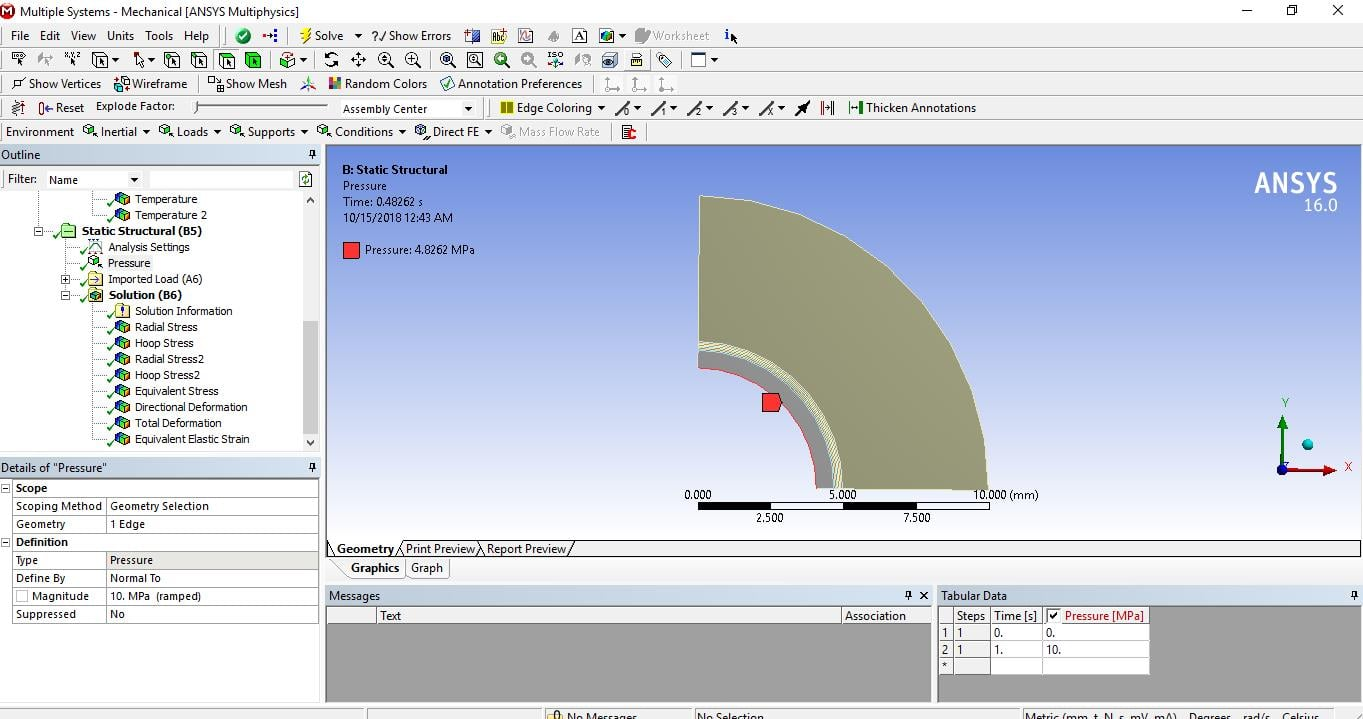1.Here i applied 10 Mpa but on my model it changes to 4.86 Mpa.

2.For my model i am getting the maximum radial stress -128.73 while i applied just 10 Mpa. Is it practically possible? I am not clear about it.The result graph is given below: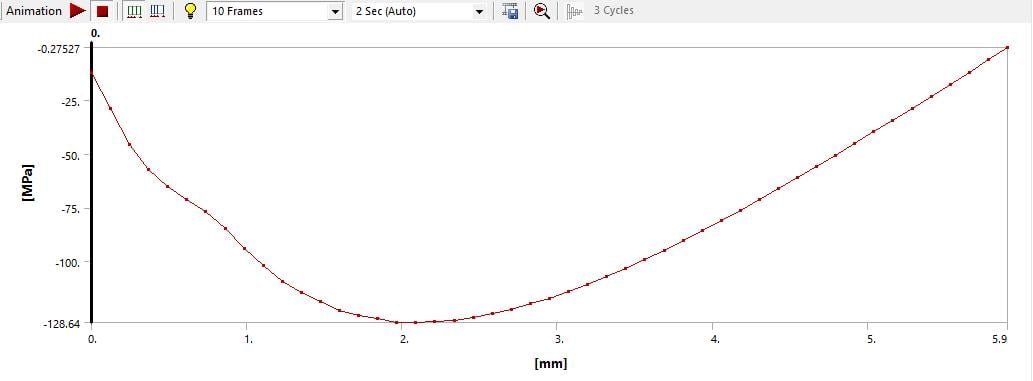3.Another thing i want to know that for my model which equations are solved to get the deformation and radial or hoop stress in Ansys. can anyone help me to understand this properly?

4. I have applied internal pressure 10 Mpa. If i  want the thermal stresses for the pressure 0 Mpa that means for the 1100 temperature only then how will i do it

• 1. The 10 MPa pressure is multiplied by the Time during the solution. When Time = 1, the Pressure = 10 MPa.  The Time in your screenshot is 0.486 therefore the applied pressure is 4.86 MPa.

3. The title of this discussion includes the words "plane strain" yet the model you have provided is using Plane Stress.

You asked about the difference between Plane Stress and Plane strain on April 25

I told you on May 18 that you have to use Plane Strain in your Static Structural model and how to do that. This is important because the equations for Plane Stress assume the Z component of stress is zero, while in Plane Strain, the equations assume the Z component of strain is zero. These different assumptions result in different equations and different results.

• edited October 2018

Nice To see you again sir.

Ok Sir i am correcting the model i made. And coming again with new questions.

Thank you very much.

• edited October 2018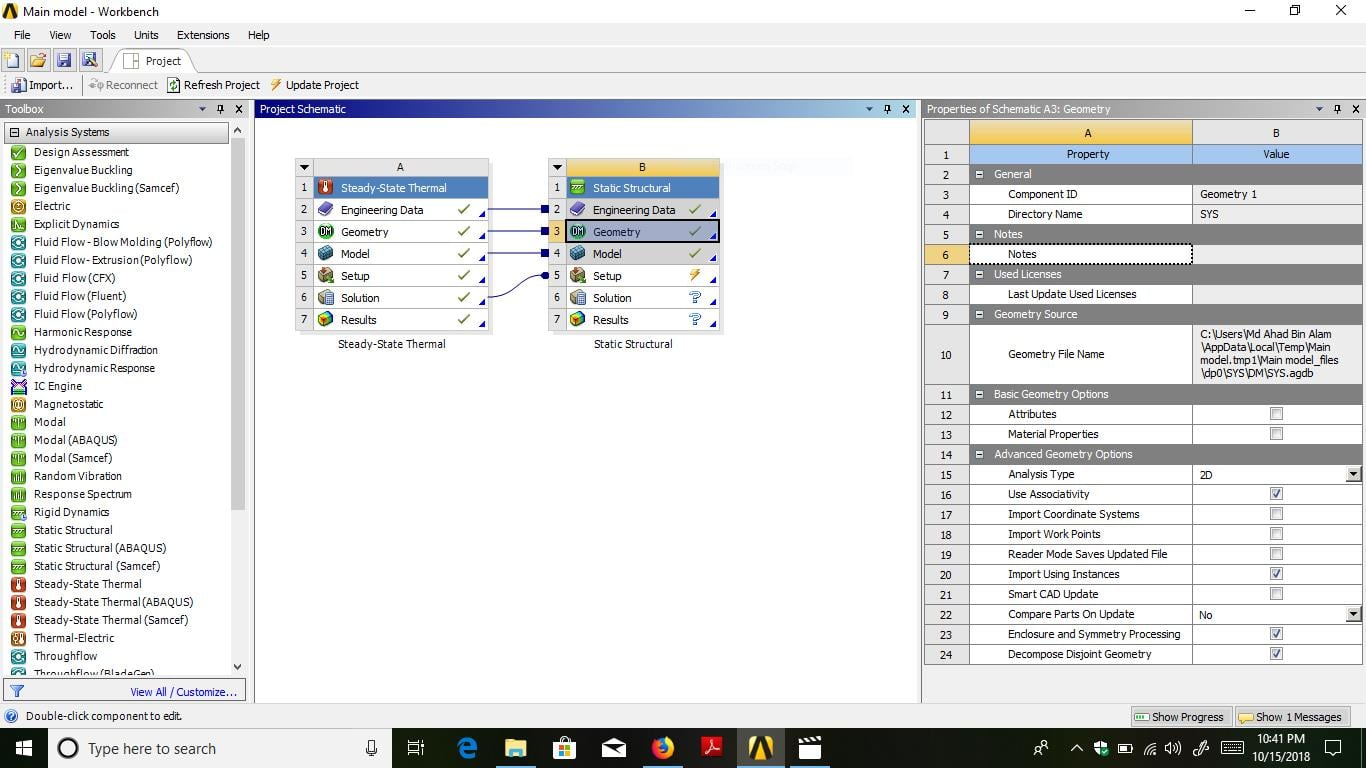1. Sir From where i can select plane strain?

•  First duplicate the Structural Model and link the Thermal Solution to the Structural Setup.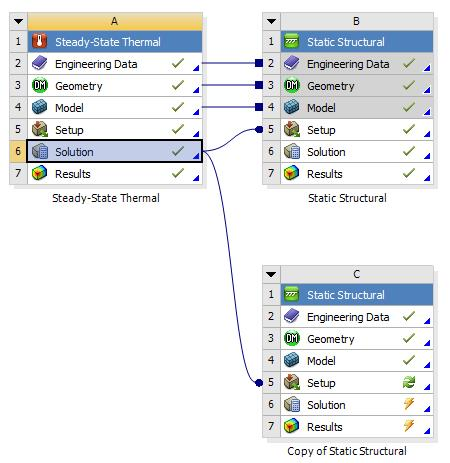Open the Structural Model of system C. Click on the Geometry branch and change the 2D Behavior.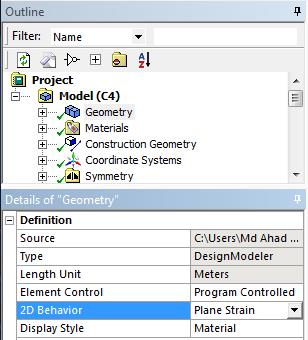• edited October 2018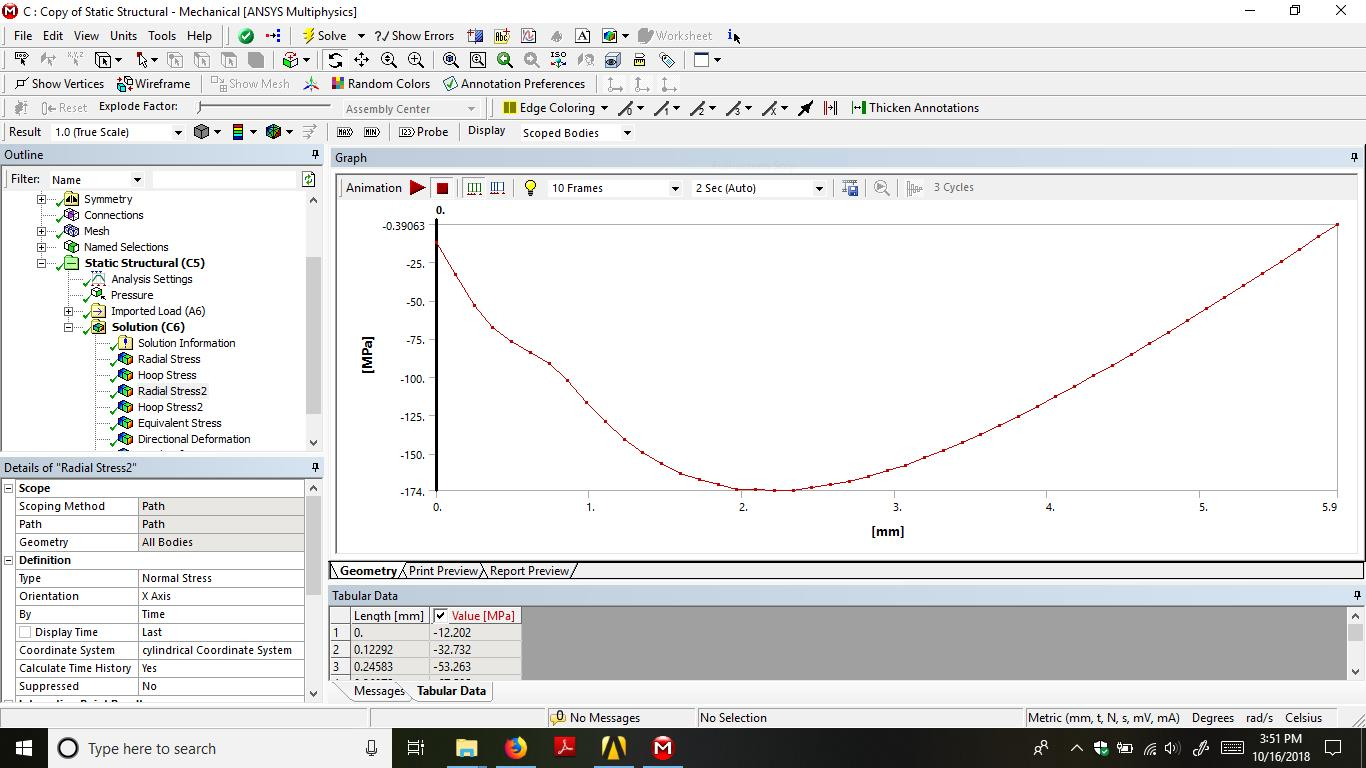1. Sir i have changed geometry into plane strain. But here there is only one load applying that is the pressure. But my aim is to analyze the thermoelastic characteristics. So i want to apply temperature as well as pressure too in this model.

2. Are the results (stresses) we got from my model valid ? i am just tensed about it. It would be very helpful if you can assure me about the radial stress, hoop stress i get from my model are ok.

3. Sir i made a 3 layered homogeneous 2D cylinder to validate my model with the analytical result. Can you help me checking whether my ansys model is ok with the analytical result. I am attaching the 3 layered homogeneous 2D cylinder model with this reply and the link of the analytical code from mathematica is gievn below:

https://1drv.ms/u/s!AnpU0q59bvps8jy-ERbH743CCdBy

I want to validate my model sir.

And thank you very very much sir. You have helped me a lot. I have learnt so many things from you

Regards.

• I found two websites with thick cylinder stress calculators on them.
I suppressed the Thermal Load and changed all three materials to middle.
Both calculators showed that the radial, hoop and axial stress for a single material cylinder are correctly computed by ANSYS (no surprise).
Your mesh is coarse, so your results have a small error compared with the exact solution.
If you reduce the radial thickness of your elements, you will reduce the size of the error.

I don't have mathematica so I can't look at the equations for the 3-layer cylinder.
It is possible to write the equations for a 3-layer thick cylinder. I would use MathCAD.
If you don't have that you could use Excel, but that is harder to find mistakes in coding.

• edited October 2018

Sir

1. I have attached the pdf file link here of the mathematica code of the 3 layered homogenous 2d cylinder i mentioned before. You can check what i tried to make into the ANSYS. Were my assumption in the Ansys model according to the mathematica code or not !

https://1drv.ms/b/s!AnpU0q59bvps8j1tKXleBXvWOti6

2. Sir if i want to get the stress for the temperature only then how can i get it from my model. I tried to apply in the steady state thermal then i can get the stresses. Then i tried in the static structural ,there i applied thermal condition and applied no pressure.Then i got stresses. Is it okay? I meant if i want to apply 0 internal pressure and 1100 deg cel internal , 100 outer temp and then want to get the stresses then how will i do it?

.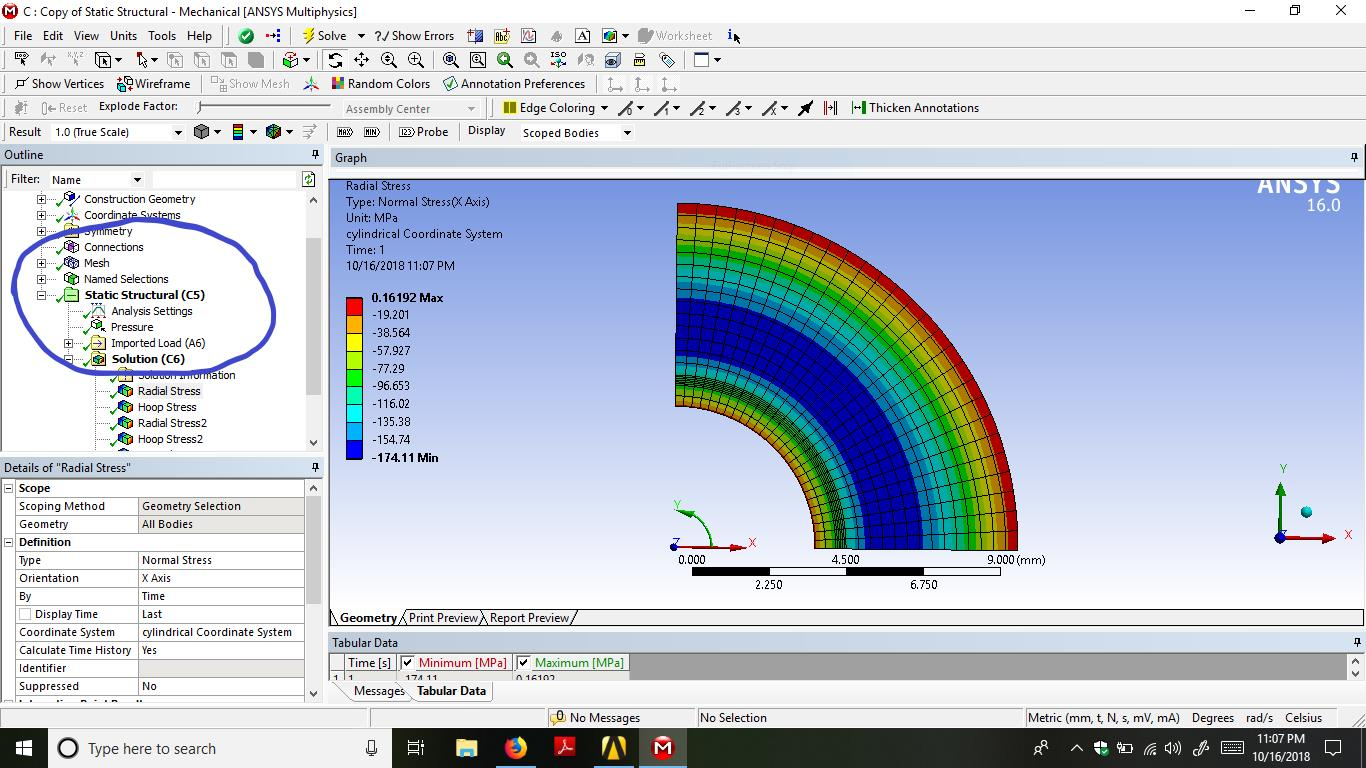3.sir when i linked as you told earlier for the plane strain then i got the stresses for pressure only. There is no temperature applied. But i want thermoelastic characteristics that means the stresses for the temperature and pressure simultaneously. then how will i do it.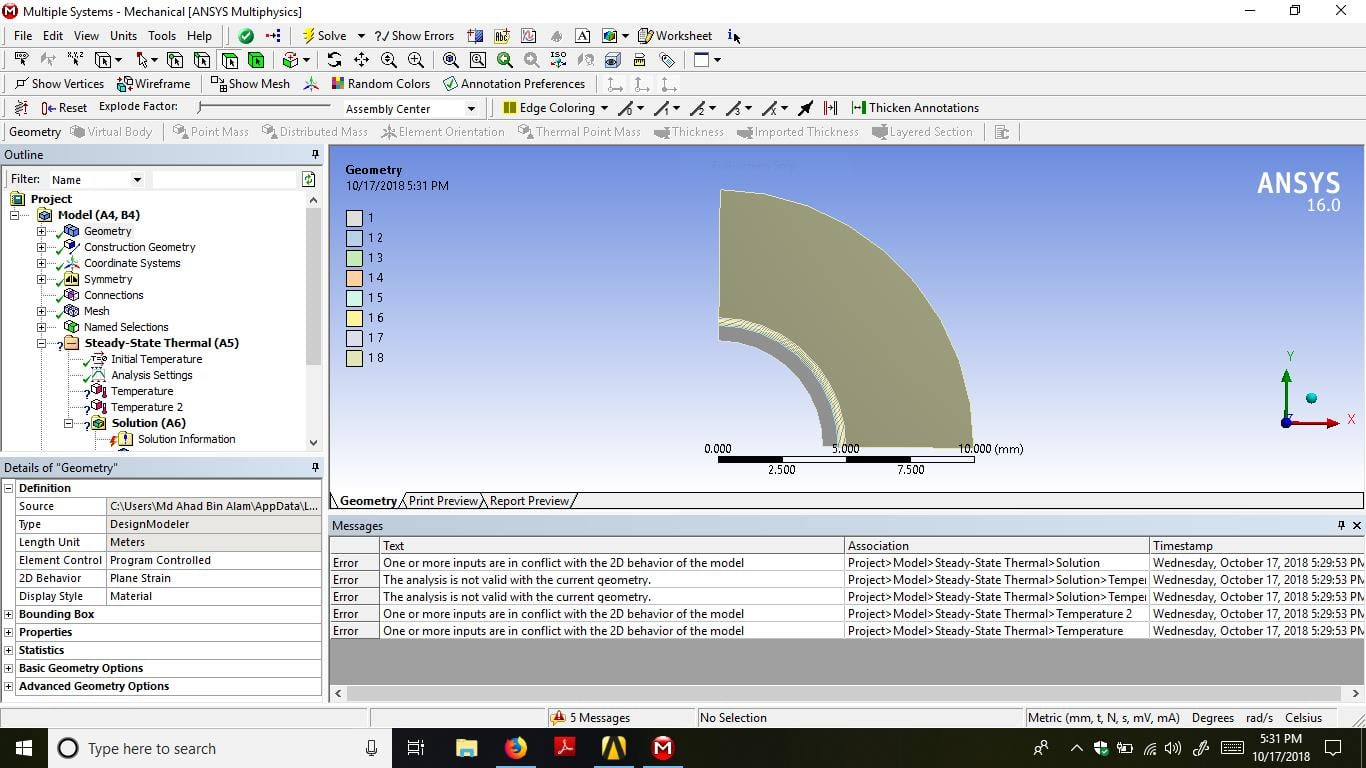sir when i tried thermoelastic characterstics that means pressure and temoerature both are applied at the same time then i got these errors.

• edited October 2018

Sir,

I need you help regarding temperature apply problem in my plane strain geometrical model.

• 1. I'm not going to validate your mathematica code.

2. If I suppress the Pressure in your 3-layer model, the Static Structural model solves for the thermal stress without error. In your Main model, you have the stress from both the pressure and the thermal load in the Copy of Static Structural system C.  You don't need system D, Final one.

3. In Copy of Static Structural system C, you have both Pressure and an Imported Temperature load. Why do you say there is no temperature applied?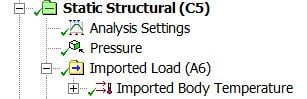I don't know where the last image in your post came from. The Main model has solved correctly.